### Type I SS and Estimable Functions

In PROC GLM, the Type I SS and the associated hypotheses they test are byproducts of the modified sweep operator used to compute a generalized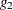-inverse ofand a solution to the normal equations. For the model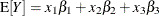, the Type I SS for each effect are as follows:

Effect

Type I SS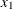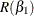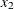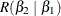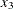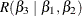Note that some other SAS/STAT procedures compute Type I hypotheses by sweeping(for example, PROC MIXED and PROC GLIMMIX), but their test statistics are not necessarily equivalent to the results of using those procedures to fit models that contain successively more effects.

The Type I SS are model-order dependent; each effect is adjusted only for the preceding effects in the model.

There are numerous ways to obtain a Type I hypothesis matrixfor each effect. One way is to form thematrix and then reduceto an upper triangular matrix by row operations, skipping over any rows with a zero diagonal. The nonzero rows of the resulting matrix associated withprovide ansuch that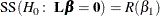The nonzero rows of the resulting matrix associated withprovide ansuch that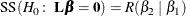The last set of nonzero rows (associated with) provide ansuch that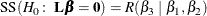Another more formalized representation of Type I generating sets for,, and, respectively, is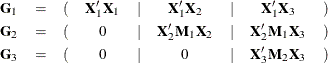where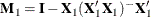and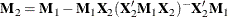Using the Type I generating set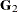(for example), if anis formed from linear combinations of the rows ofsuch thatis of full row rank and of the same row rank as, then SS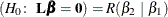.

In the GLM procedure, the Type I estimable functions displayed symbolically when the E1 option is requested are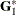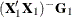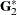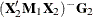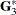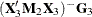As can be seen from the nature of the generating sets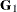,, and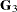, only the Type I estimable functions for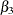are guaranteed not to involve the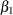and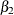parameters. The Type I hypothesis forcan (and often does) involveparameters, and likewise the Type I hypothesis foroften involvesandparameters.

There are, however, a number of models for which the Type I hypotheses are considered appropriate. These are as follows:

• balanced ANOVA models specified in proper sequence (that is, interactions do not precede main effects in the MODEL statement and so forth)

• purely nested models (specified in the proper sequence)

• polynomial regression models (in the proper sequence)